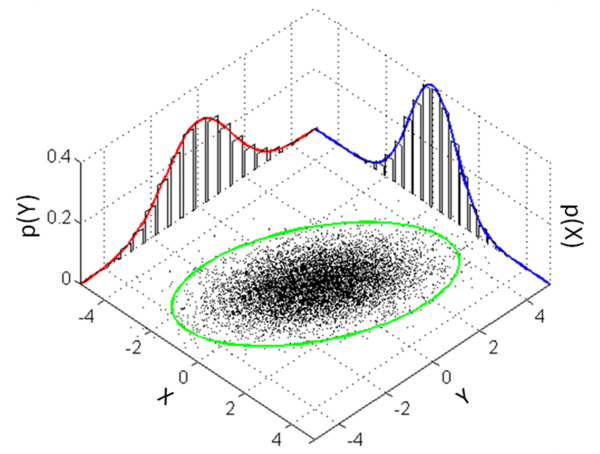##### What is marginal distribution?

The marginal variables are those variables that will be retained in the subset of variables. To find these concepts, sum The values in a table arranged …

Dec. 3, 2022 1 minuteThe marginal variables are those variables that will be retained in the subset of variables. To find these concepts, sum The values in a table arranged in rows and columns, and write the sum in the table's margins.

In marginal statistics, the distribution of the marginal variables concerning the distribution of the variables being discarded is marginalized (That is, concentrating on the margin amounts).

It is possible to begin with, a set of random variables, extend it by defining new ones (such as the sum of the originals), and then reduce the number by examining the marginal distribution of a subset (for example, the sum).

Marginal variables can be treated in various ways, each using a different subset of variables.

## How to find marginal distribution?

You completely ignore the other variable if you're asked to find marginal distribution for one of two variables. Determine how the remaining variables are distributed, assuming it does not exist.

## How to calculate marginal distribution?

Description of a marginal distribution = If X and Y are discrete random variables and f (x,y) is the value of their joint probability at (x,y), the functions delivered by: g(x) = Σy f (x,y) and h(y) = Σx f (x,y) are the marginal distributions of X and Y, respectively (Σ = summation notation). That's probably all you need to know if you're good at equations.## Conclusion

Marginal distributions are probability distributions of subsets of a collection of random variables. In the subset, there are probabilities for different values of the variables without reference to other variables.

A conditional distribution, on the other hand, provides probabilities based on other variables' values.